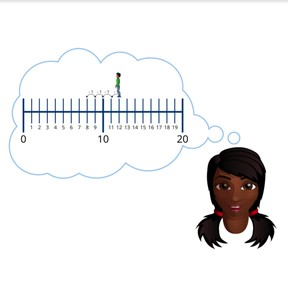Subtraction to 20 on the number line, crossing ten

# Subtraction to 20 on the number line, crossing ten

No account needed.8,000 schools use Gynzy92,000 teachers use Gynzy1,600,000 students use Gynzy

## General

Students learn to subtract numbers to 20, making use of the number line and crossing ten.

1.OA.C

## Relevance

It is important to know how to subtract, so you know how many you have left when something is taken away.

## Introduction

The interactive whiteboard shows a few subtraction problems, in which the first number (minuend) is always ten. One of the remaining numbers is hidden under a paint splatter. Students must determine what the missing number is.

## Development

Start by discussing the image of the apples with the students. How can they determine how many apples are still in the tree after 5 fell out? Next, walk students through the three steps of solving a subtraction problem using the number line. First students must look at the first number (minuend) of the subtraction problem and move to that number on the number line. Secondly, students must look at the second number (subtrahend) of the subtraction problem to determine how many steps they must jump back on the number line. Remind students that it can be useful to first jump to 10 and then jump on. This means you must be comfortable decomposing the second number (subtrahend). The third step is to determine which number they end at, which is their difference. To check that students can subtract using the number line, ask students to complete the subtraction problems given on the interactive whiteboard as a class. Remind students that they can choose how big to make their jumps back on the number line (by one, two, etc) and that they should first jump to 10, and then on.

To check that students understand subtraction to 20 on the number line crossing ten, you can ask the following questions:
- Which number in the subtraction problem tells you where you start on the number line?
- Which number in the subtraction problem tells you how many jumps back you need to take?
- What is a useful first jump?
- How do you know what the difference is?

## Guided practice

Students start by doing a subtraction problem on a number line with all the numbers marked. They are then asked to solve using a number line that only shows the jumps, and finally students must determine both the jumps and the difference.

## Closing

Discuss why it is important to be able to subtract with numbers to 20. The interactive whiteboard shows three different subtraction problems and three number lines. Students must determine which subtraction problem matches each number line. Ask students how they determined which match. Point out that students must look at the first number in the subtraction problem (minuend) to determine where to start on the number line. Solve the problems as a class.

## Teaching tips

Students who have difficulty with this learning goal can be supported by refreshing the number line to 20. They can also be supported by refreshing the decompositions of numbers to 20 so they can calculate to 10 and then continue subtracting. You can practice by giving them number cards from 1 to 9 and asking them to pair the cards that form ten.

## Instruction materials

optional: number cards 1-9

### The online teaching platform for interactive whiteboards and displays in schools

• Save time building lessons

• Manage the classroom more efficiently

• Increase student engagement